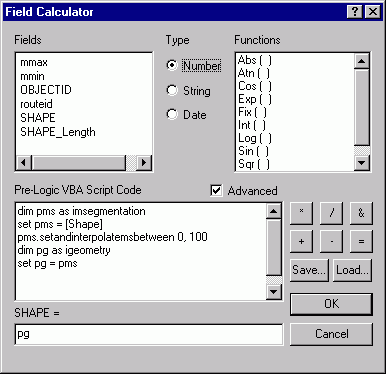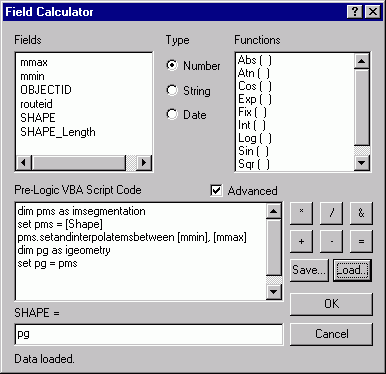English

# How To: Edit measure (M) values with the Field Calculator

## Summary

Use the ArcMap Field Calculator to calculate the measure values for the geometry of a polyline feature.

## Procedure

Familiarity with the ArcObjects Geometry system is required before attempting this method of setting M values.

1. Start editing a polyline feature class.
2. Select one or more features.
3. Open the feature class attribute table.
4. Right-click the SHAPE field and select Calculate Values.
5. In the Field Calculator, check the Advanced check box.
6. Enter the VBA expression
```dim pms as imsegmentation
set pms = [Shape]
pms.setandinterpolatemsbetween 0,100
dim pg as igeometry
set pg = pms```
The example shows how to calculate the M values to range between 0 and 100 for a selected set of polylines.This example shows how to calculate the M values to range between the values stored in two items for a selected set of polylines.```Note:
It is also possible to edit the M values for a polyline feature by using the Edit Sketch Properties dialog. See the Related Link below. ```